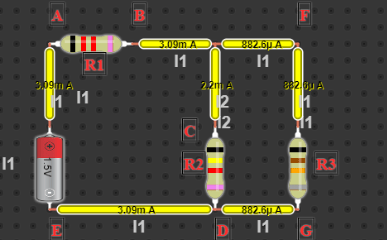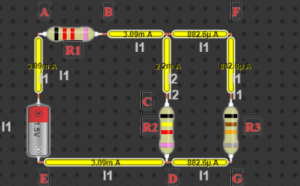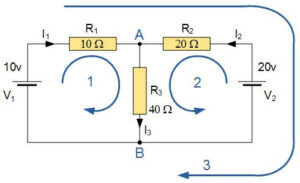# Analysis of Kirchhoff’s Circuit Laws and Simulation

Introduction

Kirchhoff’s circuit laws are central to circuit analysis. We have the basic tool to begin analyzing circuits with the help of these laws and the equations for individual components (resistor, capacitor, and inductor). In this article, we’ll look at Kirchhoff’s current and voltage laws and how they can be used in circuit analysis.

In the lumped element model of electrical circuits, Kirchhoff’s circuit laws are two equalities that deal with current and potential difference, or voltage. German physicist Gustav Kirchhoff first wrote about them in 1845. This came before James Clerk Maxwell’s study and generalized Georg Ohm’s findings. They are often referred to as Kirchhoff’s laws or simply Kirchhoff’s laws and are widely utilized in electrical engineering. These rules serve as the foundation for network analysis and are applicable in both the time and frequency domains.

Why Kirchhoff’s Laws Need

Kirchhoff’s laws can be used to calculate unknown values in a circuit, such as current and voltage. These principles are helpful for locating the unknown values in intricate circuits and networks and can be applied to any circuit (with some restrictions). To determine the values of current and voltage, it is also employed in nodal and mesh analyses. Applying KVL (each loop) to compute the current through each independent loop, and counting all the current to determine the current through any component of a circuit (Applicable in Loop Current Method). For a better knowledge of how energy moves across an electric circuit, consult Kirchhoff’s Laws.

Design of Kirchhoff’s Law circuit

Voltage and current measurements are taken from a series-parallel circuit in this experiment. After that, the Kirchhoff’s loop and junction law is validated by comparing the theoretical calculation to actual voltage and current measurements.

Equipment and Materials:

• Power Supply
• Multimeter
• Circuit Board
• 7 wires for connecting
• 3 resistor pieces (200, 400, 1K) Ω

Circuit Design:

1.Figure 1: Kirchhoff’s Law Circuit

Procedure of Design of Kirchhoff’s Laws Circuit

Part 1 Junction Law:

1. Turn on the power supply unit and set the voltage to 9.0 volts. V 0 will have this value.
2. Build the circuit depicted in the figure above and link it to the power supply unit. It is worth noting that the resistors R1, R2, and R3 create a series-parallel combination.
3. Select ammeter mode on the multimeter. The ammeter should only be connected in series to the circuit for this section.
4. Connect the ammeter to point B with the right polarity and record the result. Measure the points C, D, E, F, and G again.

Part 2 Loop Law:

1. Insert the multimeter into voltmeter mode. The voltmeter should only be connected to the circuit in parallel for this part.
2. Connect the voltmeter with the right polarity across points A and B using the same circuit as in Part I and note the results.
3. Measurements should be repeated at sites C, D, and then F, G, respectively.

Analysis of Kirchhoff’s Law Circuit

First Law of Kirchhoff’s The Current Law (KCL)

According to Kirchhoff’s Current Law (KCL), “the total current or charge entering a junction or node is precisely equal to the charge exiting the node since it has nowhere else to go, as no charge is wasted within the node.” In other words, I(exiting) + I(entering) = 0; the algebraic total of ALL currents entering and leaving a node must be equal to zero. Kirchhoff’s theory is frequently referred to as the conservation of charge.

I1 + I2 = I1

I1 + I2=I1Figure 2: KCL

Second Law of Kirchhoff’s Voltage Law (KVL)

Also known as his Second Law of The Voltage, asserts that “in any closed loop network, the total voltage around the loop is equal to the sum of all the voltage drops within the same loop,” which is also equal to zero. In other words, all of the voltages inside the loop’s algebraic total must be zero. Kirchhoff’s theory is referred to as the Conservation of Energy.Figure 3: KVL

Kirchhoff’s Circuit Laws are central to DC circuit analysis. We have the basic tool to begin analyzing circuits with the help of these laws and the equations for individual components (resistor, capacitor, and inductor). In this article, we’ll look at Kirchhoff’s current and voltage laws and how they can be used in circuit analysis. Kirchhoff’s Circuit Laws enable us to solve complex circuit problems by defining a set of fundamental network laws and theorems for voltages and currents in a circuit. We can calculate and find the currents and voltages around any closed-circuit using Kirchhoff’s circuit law relating to the junction rule and his closed loop rule if we know the values of the electrical components within it. We saw in the Resistors tutorial that when two or more resistors are connected in series, parallel, or combinations of both, a single equivalent resistance (RT) can be found, and that these circuits obey Ohm’s Law. However, in complex circuits such as bridge or T networks, we cannot always rely solely on Ohm’s Law to determine the voltages or currents circulating within the circuit.

Kirchhoff’s Circuit Laws Terms:

1. Circuit: A circuit is a closed loop conducting path through which an electrical current flow.
2.  Path: A single line connecting two or more elements or sources.
3. Node: A node is a junction, connection, or terminal within a circuit where two or more circuit elements are connected or joined together to provide a connection point between two or more branches. A dot represents a node.
4. Branch: A branch is a single or group of components connected between two nodes, such as resistors or a source.
5. Loop: A loop is a simple closed path in a circuit that encounters no circuit element or node more than once.
6. Mesh: a mesh is a single closed loop series path that is devoid of all other paths.

Example of Kirchhoff’s Circuit Law

R3’s 40 Resistor current flow should be determined. The circuit has 3 branches, 2 nodes (A and B) and 2 independent loops. The equations are presented below using Kirchhoff’s Current Law, abbreviated KCL:

I1 + I2 = I3 at node A

I3 = I1 + I2 at node B

The equations are presented below using Kirchhoff’s Voltage Law, abbreviated KVL:

1st loop as follows: R1 I1 + R3 I3 = 10I1 + 40 I3.

Given is the 2n loop: R2 I2 + R3 I3 = 20 = 20I 2 + 40 I3

Given is the loop 3: 10 – 20 = 10 I1 – 20 I2

We may rewrite the equations as follows: Eq. No. 1: 10 = 10I1 + 40(I1 + I2) = 50 I1 + 40 I2 since I3 is the product of I1 and I2.

Equation number two: 20 = 20 I2 + 40 (I1 + I2) = 40 I1 + 60 I2

Use online circuit simulators to simulate and experiment with Kirchhoff’s circuit laws, online circuit simulators can make understanding Kirchhoff’s circuit laws easier. Try the virtual lab from DCACLab for free and click on the analysis tool button to show all loops and formulas.

Kirchhoff’s Circuit Laws are used Example 2:

Kirchhoff’s Circuit Laws are used in the following manner to find the currents and voltages in a circuit, which is referred to as “analyzing” the circuit.

1. Assume that all resistances and voltages are known. If not, write V1, V2, R1, R2, etc. on them.
2. Distributes a current (clockwise or counterclockwise) to each branch or mesh.
3. Add a branch current to each branch. I1, I2, I3, etc.
4. Discover the equations for each node’s Kirchhoff’s first law.
5. Determine the Kirchhoff’s second law equations for each of the circuit’s independent loops. Use linear simultaneous equations to locate the elusive currents as needed.

Simulation of Kirchhoff’s Circuit LawsFigure 4: Simulation of Kirchhoff’s Circuit Laws

Conclusion:

The different voltages and currents flowing through a linear circuit may be calculated using Kirchhoff’s Circuit Law, but we can also utilize loop analysis to determine the currents in each separate loop, which helps to decrease the amount of mathematics needed compared to using only Kirchhoff’s rules. To achieve that, we’ll look into mesh current analysis in the upcoming course on DC circuits.

Md. Anisur Rahman

•
•
•
•
•
•

#### Article written by: Md. Anisur Rahman

I am Md. Anisur Rahman Likhon. I have completed Cyber Security for Masters of Science in Computer Science & Engineering (MSCSE) at United International University in 2022. After passing the S.S.C examination from Melandah Umir Uddin Pilot High School in 2010 I got admitted to Habibur Rahman College Mahmudpur and I have completed HSC in 2012. I have completed a Bachelor of Social Science (BSS) Hon's in Bangladesh Open University (BOU) in 2018. I have completed Post Graduate Diploma in Information Technology (PGDIT) from IIT, Jahangirnagar University in 2020. Now I am being a job as IT Support Staff at United International University. I am both Technical Content Writer & Instructor at Electronics Circuit Design in DCAClab.com.